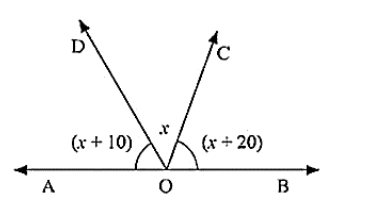# In the given below figure, find x. Further find

Question:

In the given below figure, find x. Further find ∠COD, ∠AOD, ∠BOCSolution:

Since ∠AOD and ∠BOD form a line pair,

∠AOD + ∠BOD = 180°

∠AOD + ∠BOC + ∠COD = 180°

Given that,

∠AOD = (x + 10)°, ∠COD = x°, ∠BOC = (x + 20)°

(x + 10) + x + (x + 20) = 180

3x + 30 = 180

3x = 180 - 30

3x = 150/3

x = 50

Therefore, ∠AOD = (x + 10)

= 50 + 10 = 60

∠COD = x = 50°

∠COD = (x + 20)

= 50 + 20 = 70

∠AOD = 60°∠COD = 50°∠BOC = 70°